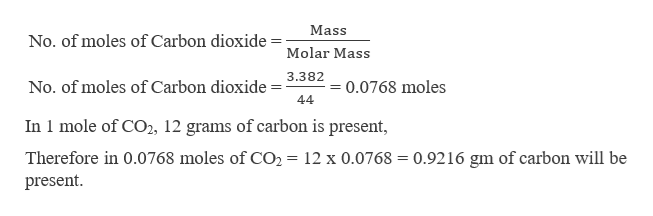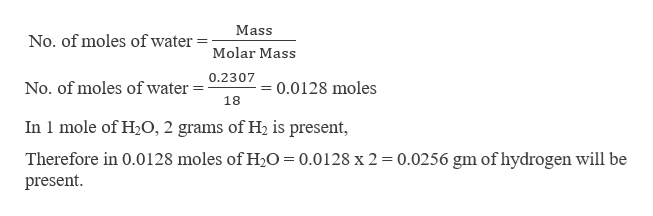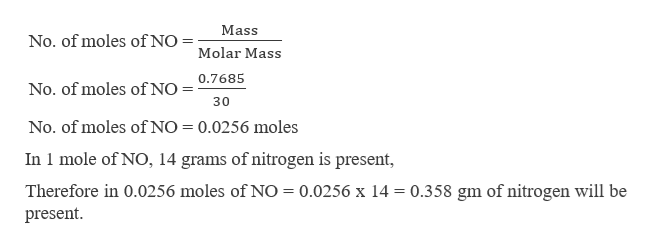# A sample of a substance (containing only C, H, and N) is burned in oxygen.3.382 g of CO2, 2.307×10-1 g of H2O and 7.685×10-1 g of NO are the sole products of combustion.What is the empirical formula of the compound?

Question
6 views

A sample of a substance (containing only C, H, and N) is burned in oxygen.
3.382 g of CO22.307×10-1 g of H2O and 7.685×10-1 g of NO are the sole products of combustion.
What is the empirical formula of the compound?

check_circle

star
star
star
star
star
1 Rating
Step 1

To calculate the empirical formula, it is required to calculate the amount of carbon, hydrogen and nitrogen present in the sample. This can be done as,help_outlineImage TranscriptioncloseMass No. of moles of Carbon dioxide Molar Mass 3.382 0.0768 moles No. of moles of Carbon dioxide 44 In 1 mole of CO2, 12 grams of carbon is present, Therefore in 0.0768 moles of CO2 = 12 x 0.0768 = 0.9216 gm of carbon will be present fullscreen
Step 2

Calculating the mass of Hydrogen,help_outlineImage TranscriptioncloseMass No. of moles of water Molar Mass 0.2307 0.0128 moles No. of moles of water 18 In 1 mole of H2O, 2 grams of H2 is present, Therefore in 0.0128 moles of H20 = 0.0128 x 2 0.0256 gm of hydrogen will be present fullscreen
Step 3

Calculating the mass...help_outlineImage TranscriptioncloseNo. of moles of NOMolar Mass Mass No. of moles of NO = 0.7685 30 No. of moles of NO 0.0256 moles In 1 mole of NO, 14 grams of nitrogen is present, Therefore in 0.0256 moles of NO 0.0256 x 14 0.358 gm of nitrogen will be present. fullscreen

### Want to see the full answer?

See Solution

#### Want to see this answer and more?

Solutions are written by subject experts who are available 24/7. Questions are typically answered within 1 hour.*

See Solution
*Response times may vary by subject and question.
Tagged in

### General Chemistry